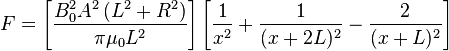# Force of attraction between two bar magnets

NMavro
Hi,

I have carried out an experiment to investigate the force of attraction between two bar magnets by changing the separation between the two magnets. Both bar magnets are about 4cm long and I have recorded results between 1cm and 10cm's.

A lot of the research I have carried out appears to say that the force between two magnets cannot be calculated at a close distance and that for large distances, is this true? It also says I should use the force of attraction from the two further poles and the repulsion force from the like poles in my general formula. However, as distance increases, wouldn't that make those negligible as the force is inversely exponential as distance increases.

Could you please clarify those problems and also tell me the exact relationship between force and distance is in this case?

Kind regards,
NMavro

NMavro
Hi, thank you for you quick reply Electric Red. However, I'm afraid it does not. What I meant to say was, what would be considered a large distance for bar magnets of 4cm's of length. I am also trying to find the exact relationship between the force of attraction of two bar magnets and their separation.

Gold Member
Hi, thank you for you quick reply Electric Red. However, I'm afraid it does not. What I meant to say was, what would be considered a large distance for bar magnets of 4cm's of length. I am also trying to find the exact relationship between the force of attraction of two bar magnets and their separation.

We went over this last year:

I was just trying to give you some idea of how much force you would need for a a quick (but controlled) deceleration.

Force between magnets - Wikipedia, the free encyclopedia

Yay! I've in the past, wondered the same thing; "Why don't they just put magnets on bumpers?"

And thank you for that link Aleph. An equation from that linkI :!) PF!I would explain what all the terms in the equation are, but I'm late for work. Click on Aleph's link, and they will be explained.

Ciao!

NMavro
Thanks, however, I am still confused.

I agree with this formula, but I am not quite sure as of how this applies to large distances. As it can be seen in this formula, there are three parts. In the formula above, x is the separation between the two magnets and L is the length of the magnets.

The first one, with 1/x^2 shows the force of attraction between the two closest opposite poles.
The second one, 1/(x + 2L)^2 is the attraction force between the furthest opposite poles.
Finally, the repulsion force must be removed which is shown by - 2/(x + L)^2.

Below this formula on Wikipedia, it says ''Note that all these formulations are based on the Gilbert's model, which is usable in relatively great distances.''. What I am wondering is what is considered a ''great distance'' and why at a great distance the second and third part of the equation are not negligible as their separations are a lot bigger and the force is proportional to the inverse square of the separation.

Gold Member
Thanks, however, I am still confused.

I agree with this formula, but I am not quite sure as of how this applies to large distances. As it can be seen in this formula, there are three parts. In the formula above, x is the separation between the two magnets and L is the length of the magnets.

The first one, with 1/x^2 shows the force of attraction between the two closest opposite poles.
The second one, 1/(x + 2L)^2 is the attraction force between the furthest opposite poles.
Finally, the repulsion force must be removed which is shown by - 2/(x + L)^2.

Below this formula on Wikipedia, it says ''Note that all these formulations are based on the Gilbert's model, which is usable in relatively great distances.''. What I am wondering is what is considered a ''great distance'' and why at a great distance the second and third part of the equation are not negligible as their separations are a lot bigger and the force is proportional to the inverse square of the separation.

Wow. Thank you for making me stop and think about that equation. I'd originally used it to determine the "face flux" value of my magnets. I never really stopped to look at the 3 terms. When x>>>>>L, the equation reduces to 0/x2

I hooked up an experiment this morning with 4 cm long magnets, but I decided I could not determine anything useful, as my house is a menagerie of stray magnetic flux fields.

I have nails in the floor, 70's era speakers in all four corners of my living room, and the stupid Earth's magnetic field to contend with.

I gave up, as I had to get to work, and the distances were so large, that everything oscillated for minutes. :grumpy:

Last edited: